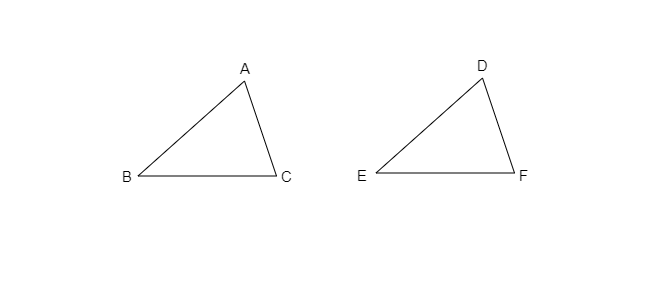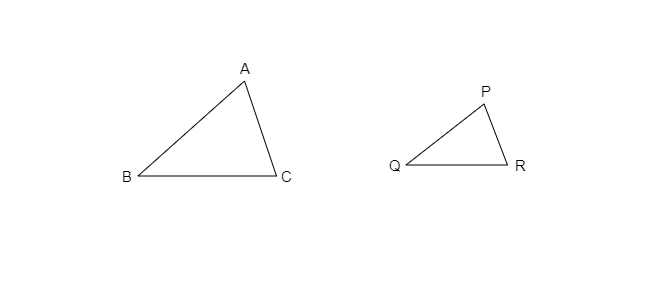# In a squared sheet, draw two triangles of equal areas such that$(i)$. the triangles are congruent.$(ii)$. the triangles are not congruent.What can you say about their perimeters?

To do: To draw two triangles of equal areas such that

$(i)$. the triangles are congruent.

$(ii)$. the triangles are not congruent.

To compare the perimeters of two triangles if they are congruent and not congruent.

Solution:

$(i)$. If two triangles are congruent, then all the corresponding parts of the triangles will be equal.

Let us consider two triangles, $ΔABC$ and $ΔDEF$On the given square sheet, we have drawn two congruent triangles.

such that, $∆ ABC ≅ ∆ DEF$

In a squared sheet, draw two triangles of equal areas such that (i) the triangles are congruent (ii) the triangles are not congruent What can you say about their perimeters?

We can say that,

$AB=DE$,

$BC=EF$

$AC=DF$

On adding the three relations a bove, we get

$AB+BC+AC=DE+EF+DF$

Thus, Perimeter of $∆ ABC=$ Perimeter of $∆ DEF$

$(ii)$.  In this case, we have drawn two triangles ABC and PQR which are not congruent.Such that

$\overline{AB}≠\overline{PQ}$

$\overline{BC}≠\overline{QR}$

$\overline{CA}≠\overline{RS}$

Adding the given relations, we get

$AB+BC+AC≠PQ+QR+PR$

Thus, Perimeter of $∆ ABC≠$Perimeter of $∆ PQR$.

From the above, we can say that if two triangles are not congruent then the perimeter is also not equal and if they are congruent then their perimeters are also equal.

Updated on: 10-Oct-2022

177 Views